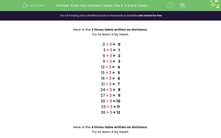# Know Division Facts for the 3 Times Table

In this worksheet, students will recall division facts from the 3 times table.Key stage:  KS 2

Curriculum topic:   Number: Multiplication and Division

Curriculum subtopic:   Use Multiplication/Division Facts (3, 4 and 8)

Popular topics:   Multiplication worksheets, Division worksheets, Times Table worksheets

Difficulty level:#### Worksheet Overview

In this activity, we will be learning about how to use the 3 times table facts to solve division problems.

Multiplication and division are inverse operations.

This means that if you know your 3 times table, you can also divide some numbers by 3 very quickly.

Let's see how!

We know that 5 × 3 = 15.

This means that in 15, there are 3 groups of 5 or 5 groups of 3.

When we know one times table fact, we can write a family of facts by rearranging the numbers:

5 × 3 = 15

3 × 5 = 15

15 ÷ 3 = 5

15 ÷ 5 = 3

This works for all times table facts.Let's try an example using the 3 times table.

Example

What is 24 ÷ 3?

We know that 24 is in the 3 times table.

3 × 8 = 24

So, we can also say that 24 ÷ 3 = 8

Now that you've seen how to use this skill, you are ready to move on to some questions by yourself.### What is EdPlace?

We're your National Curriculum aligned online education content provider helping each child succeed in English, maths and science from year 1 to GCSE. With an EdPlace account you’ll be able to track and measure progress, helping each child achieve their best. We build confidence and attainment by personalising each child’s learning at a level that suits them.

Get started